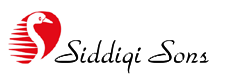T 92.21.692.3559 F 92.21.692.3640 M 0345.2825.900 E info@siddiqisons.comHomeAbout usFacilitiesPicture galleryKnowledge BaseContact usSite map

 Knowledge Base >>> Power CalculationGlossary of TermsCommon Plastics DataPlastic PropertiesShrinkage% of plasticPlastic IdentificationPower CalculationOhms law

Electrical Power

When voltage is applied to a conductor, current will flow and the amount of current flow and voltage represent a quantity of power.

Such electric power can be used for heating purposes, for operating a motor or in other applications of electric energy. Since we cannot get power for nothing, a battery or other power source must be used to generate the energy needed. The electric power symbol (P) is measured by the amount of voltage multiplied by the quantity of current flow.

P = E x I

The unit of power is the Watt, named after the Scottish inventor James Watt(1736-1819). One Watt of power is equal to one ampere of current flow produced by one volt of electric pressure.

Because the watt unit relates to electric power, the symbol VA (Volt-Amp) has been used as well as W (Watt).

When power is calculated in terms of time, the unit of energy is the Joule. This is also known as the Watt-second and represents one watt of power for one second. In the measurement of ordinary electric power consumed in homes, the KilowattHour (kWh) is utilized and this refers to 1000 Watts for 1 hour. In many electronic applications, however, only fractional power units are used, and the term milliwatt (mW) is then utilized for convience, to express one-thousandth of a watt.

Thus, 0.0005-W = 0.5 mW.

The formula P = E x I solves for the amount of energy consumed in terms of unit watts. Therfore if 20 volts are present across a resistance and 2 amps of current flow, the amount of energy consumed equals 40 watts.

If E voltage is unknown but I (amps) and R (resistance) are known, the following formula is used:

P = I² x R

Thus if 2 amps of current are flowing and the resistance is 10 ohms the amount of power is P = 2 x 2 x 10 = 40 watts.

Power can also be found by dividing the voltage squared by the resistance: P = E²/R.

Because power is related to Ohm's law, the amount of power used can be used in formulas for finding unknown values of current and voltage.

I = P/E

R = P/I²

E = ^(P x R)

I = ^(P/R)

When dealing with large amounts of electrical power, it may be required that you be able to determine the cost of the power consumed.

You will be dealing with units of kilowatts and kilowatt-hours (kWh), which means the number of kilowatts used per hour. Thus, 25 kWh is equivalent to 25 kilowatts used for 1 hour. To find the expense of an electric usage bill, the formula is:

Expense = kW x hours x rate per kWh

Voltage

V or E = I x R

As this formula shows, an unknown voltage can be found by multiplying the current by the resistance value. Thus, if the current flow through a 3 ohm resistance is 20 amps, the voltage across the resistance is 60 volts. If the value of the resistance is desired, the known values of current and voltage are used for solving the problem by use of the formula:

R = E/I or V/I

As an example, assume that the voltage across a resistance is 50 volts and the value of the current flow is 10 amps. Dividing the E value by the I value results in a resistance of 5 ohms.

Amps

I=E/R or V/R

This formula indicates that the amount of current flowing in a circuit is equal to the quotient obtained when the voltage value is divided by the resistance value. Thus, if there are 10 volts across a 5 ohm resistor, the current flow through the resistor is 2 amps.

An unknown value of either voltage or resistance can also be found by rearrangement of the formula. Voltage, for example, can be obtained if thevalues of current and resistance are known.

Because power is related to Ohm's law, the amount of power used can be used in formulas for finding unknown values of current and voltage.

I = P/E

R = P/I²

E = ^ (P x R)

I = ^ (P/R)

Ohms

If the value of the resistance is desired, the known values of current and voltage are used for solving the problem by use of the formula:

R = E/I or V/I

As an example, assume that the voltage across a resistance is 50 volts and the value of the current flow is 10 amps. Dividing the E value by the I value indicates a resistance of 5 ohms.

Because power is related to Ohm's law, the amount of power used can be used in formulas for finding unknown values of current and voltage.

I = P/E

R = P/I²

E = ^ (P x R)

I = ^ (P/R)Picture gallery | Knowledge Base | Contact us | Site map |
 © 2006 - 2010 by M/S Siddiqi Sons. This site is designed and maintained by iThoughts.com.pk